### Voronoi Diagram

Given ai, ….., aN
• Voronoi polygon (a) := all points closer to a than other ai’s.
Verteices2N-4

edges3N-6

• Voronoi diagram :=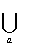polygon(a)

• Voronoi Dual (Delaunay triangulation) triangulation of all points
• Degree(vertx) = 3 (外心)

• Convex Hull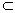Dual

• Polygon(a) unbounded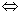convex Hull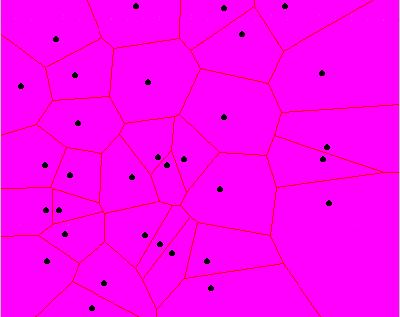# Applications

• closest pair find closest pair of ai’s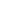(Dual)
• all nearest neighbor find nearest neighbor of ai (Dual)
• nearest neighbor find nearest ai of a point P (Ppoly(ai))
• computing convex Hull(Dual)
• Euclidean Minimum Spanning Tree(Dual)
find a tree connects all ai’s with minimum distance.
• Can compute Voronoi Diagram in O(NlogN)All can be solved in O(NlogN)

# Compute Voronoi Diagram in O(NlogN) time

(Divide & Conguer)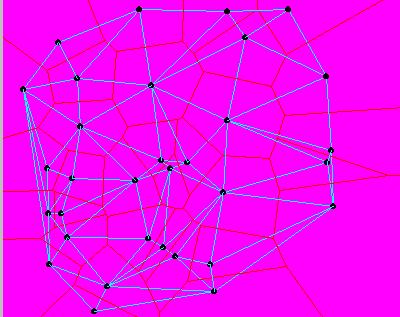VD=( VD(L)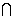L(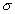) )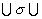( VD(R)R() )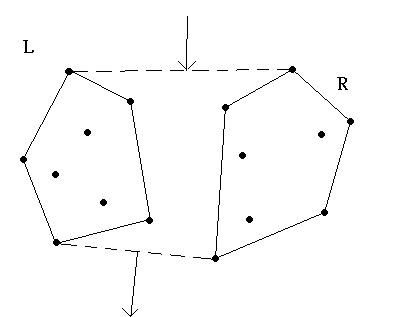# Compute Supporting Lines T=O(N)

1. Compute CH(L)CH(R)
2. Find interior point P of CH(L)
3. P interior point of CH(R)
4.5. Merge CH(L)CH(R)
6. Graham Scan (NlogN for convex Hull)

Property Pinterior point of CHAngles of vertices of CH